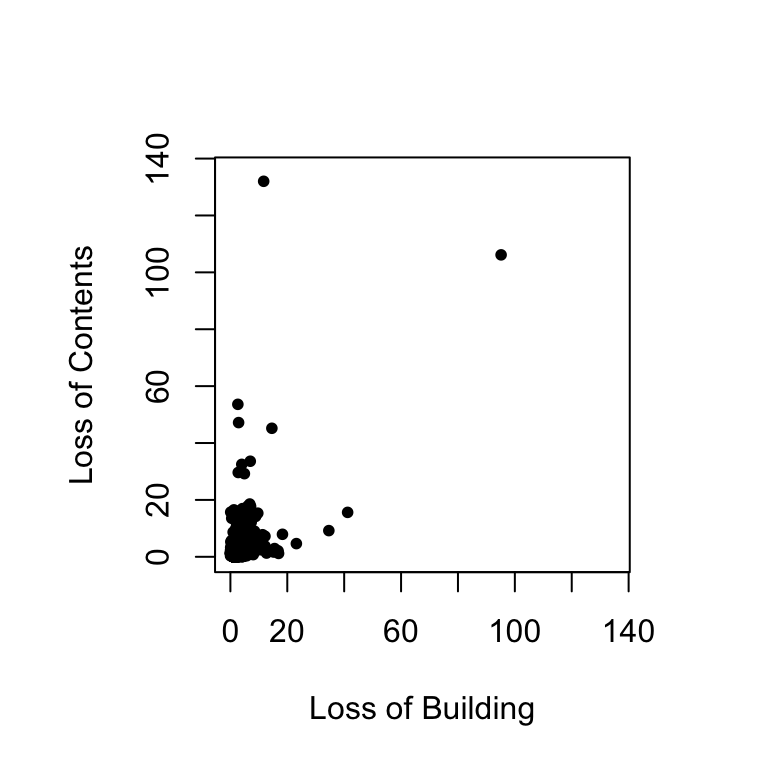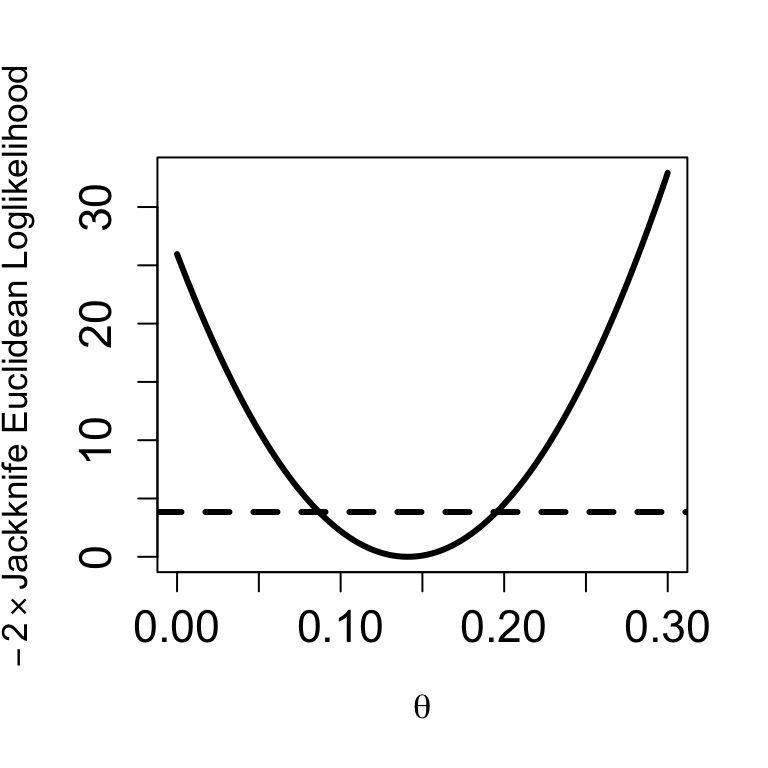Below, I discuss some R code for illustrating how to conduct jackknife Euclidean likelihood-based inferences for Spearman’s rho as proposed by de Carvalho and Marques (2012); for the interpretation of the quantities computed in this script, the reader is referred to the article. Let’s start by cleaning R workspace:

rm(list = ls())

For reproducibility reasons, I list below the information about R, the OS, and loaded packages:

sessionInfo()
## R version 3.3.2 (2016-10-31)
## Platform: x86_64-apple-darwin13.4.0 (64-bit)
## Running under: macOS Sierra 10.12.1
##
## locale:
##  en_US.UTF-8/en_US.UTF-8/en_US.UTF-8/C/en_US.UTF-8/en_US.UTF-8
##
## attached base packages:
##  stats     graphics  grDevices utils     datasets  methods   base
##
## loaded via a namespace (and not attached):
##   magrittr_1.5    assertthat_0.1  tools_3.3.2     htmltools_0.3.5
##   yaml_2.1.13     tibble_1.2      Rcpp_0.12.5     stringi_1.1.1
##   rmarkdown_1.1   knitr_1.15      stringr_1.0.0   digest_0.6.9
##  evaluate_0.10

In the code chunks below, I follow the 80 characters per line standard.

## Jackknife Euclidean Likelihood Confidence Intervals

The Danish fire insurance claims database used by de Carvalho and Marques (2012) can be downloaded from here. We are now ready to conduct the analysis.

data = read.table("danish.txt", header = T)
attach(data)

Here is how the data looks like

par(pty = "s")
plot(building, contents, pch = 20, xlim = c(0, 135), ylim = c(0, 135),
xlab = "Loss of Building", ylab = "Loss of Contents")Danish Fire Insurance Claims: Loss of Building Loss of Content

spearman <- function(b,c) {
n <- length(b)
F <- ecdf(b)
G <- ecdf(c)
return(12 / n * sum((F(b) - 1/2) * (G(c) - 1/2)))
}

s <- function(theta)
1 / n * sum((Z - theta)^2)

We start by constructing the vector of the jackknife pseudo-values:

n <- length(building)
U_n <- spearman(building, contents)
Z <- as.double()
for(i in 1:(n))
Z[i] <- n * U_n - (n - 1) * spearman(building[-i], contents[-i])

and the Euclidean loglikelihood function

e_loglike <- function(theta)
n * (U_n - theta)^2 / s(theta)

Here is a plot of the Euclidean loglikelihood function

  curve(e_loglike, from = 0, to = 0.3, type = "l", lwd = 3,
ylab = expression(paste(-2%*% "Jackknife Euclidean Loglikelihood")),
xlab = expression(theta), cex.axis = 1.4, cex.lab = 1.1)
abline(h = qchisq(0.95, 1), lwd = 3, lty = 2)Replicating Fig. 1 in de Carvalho and Marques (2012)

The Euclidean likelihood confidence intervals are obtained by identifying the points at which intersects the dashed line of the 0.95 quantile of a chi-square distribution with one degree of freedom. A simple way to perform this in R is through uniroot:

g.95 <- function(theta)
n*(U_n - theta)^2 / s(theta) - qchisq(0.95, 1)
L <- round(uniroot(g.95, interval = c(-1, U_n), tol = .1*10^{-10})$root, 4) U <- round(uniroot(g.95, interval = c(U_n, 1), tol = .1*10^{-10})$root, 4)

Thus, [0.087, 0.1952] is a 95% confidence interval for Spearman’s rho.